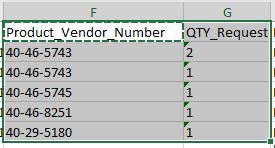# Remove duplicates from duplicates

Hello all,
I have a table that contains article numbers in column F, which can occur more than once. For the subsequent process I need the data formatted. This means that I only need the article number once and the complete total in column G. How can I map this?

As an example:
40-46-5743->Total 3HI @NHoe

``````(From d In DtBuild.AsEnumerable
Group d By k=d("Product Vendor Number").toString.Trim Into grp = Group
Let nj =  grp.Sum(Function (x) CDbl(x("QTY_Request").toString.Trim))
Let ra = New Object(){k,grp.First()(1),grp.First()(2),nj}
``````

Note : grp.First()(2) are the additional column values that need to be added

Regards
Gokul

Check out this Sample XAML file for your reference @NHoe

GroupBySumBuildDt.xaml (8.1 KB)

Regards
Gokul

your suggestion is almost perfect, however the DT_Input has 1 columns, I need the same structure back in the DT_Output.

(From d In DT_Input.AsEnumerable
Group d By k=d(“Product_Vendor_Number”).toString.Trim Into grp = Group
Let nj = grp.Sum(Function (x) CDbl(x(“QTY_Requested_for_Return”).toString.Trim))
Let ra = New Object(){k,grp.First()(1),grp.First()(17),nj}

How many column do you have in the Dt? @NHoe

You can try with this LINQ also @NHoe

``````Dt1.AsEnumerable.GroupBy(Function(r) r("Product Vendor Number").ToString).Select(Function(g) dt1.Clone.LoadDataRow({g.Key,g.Sum(Function(r) CInt(r("QTY_Request").ToString)),g.Sum(Function(r) CInt(r("").ToString))},False)).CopyToDataTable
``````

Regards
Gokul

In the Let `na` You can pass the all the column values like `grp.First()(1) -> Here (1) is the index in the excel` you can pass all the column like this.

(From d In DT_Input.AsEnumerable
Group d By k=d(“Product_Vendor_Number”).toString.Trim Into grp = Group
Let nj = grp.Sum(Function (x) CDbl(x(“QTY_Requested_for_Return”).toString.Trim))
Let ra = New Object(){k,grp.First()(1),grp.First()(17),nj}

DT_Input

DT_Out

Hi @NHoe

Try this expression and increment the column index based on the column count `grp.First()(Index)`

``````(From d In DT_Input.AsEnumerable
Group d By k=d(“Product_Vendor_Number”).toString.Trim Into grp = Group
Let nj = grp.Sum(Function (x) CDbl(x(“QTY_Requested_for_Return”).toString.Trim))
Let ra = New Object(){grp.First()(0),grp.First()(1),grp.First()(2),grp.First()(3),grp.First()(4),k,nj,grp.First()(7),grp.First()(8),grp.First()(9),grp.First()(10),grp.First()(11),grp.First()(12),grp.First()(13),grp.First()(14),grp.First()(15)}